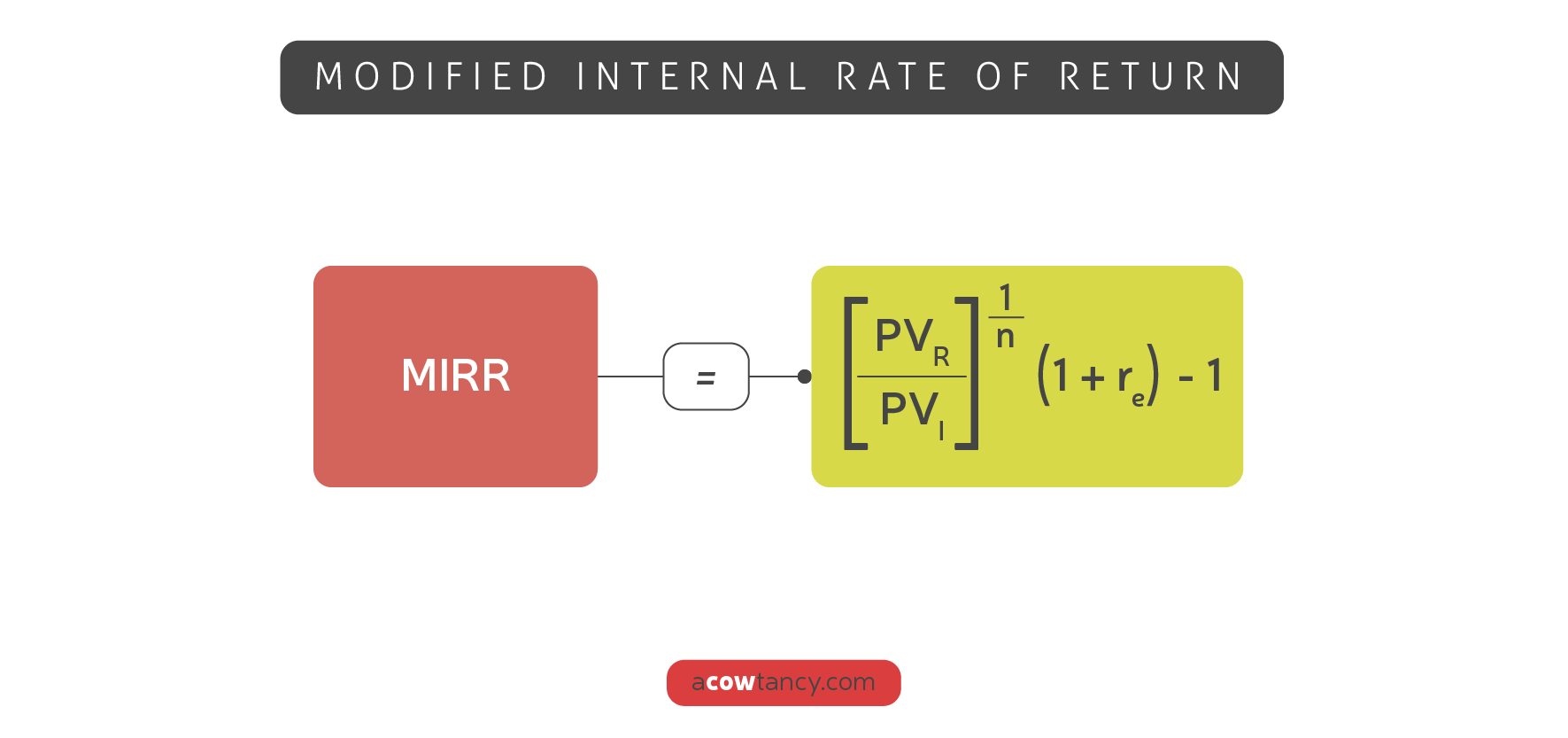AFMP4
Syllabus B. Advanced Investment Appraisal B1. Discounted cash flow techniques

# B1c. MIRR 14 / 14

### Syllabus B1c)

Establish the potential economic return (using internal rate of return (IRR) and modified internal rate of return) and advise on a project’s return margin. Discuss the relative merits of NPV and IRR.

### Modified internal rate of return gives a measure of the return from a project

MIRR = Project's return

MIRR gives a measure of the maximum cost of finance that the firm could sustain and allow the project to remain worthwhile.

If Project return > company cost of finance ⇒ Accept project

#### Calculation of MIRR

There are several ways of calculating the MIRR, but the simplest is to use the following formula which is provided on the formula sheet in the exam:#### where

• PVr= the present value of the "return phase" of the project
PVi = the present value of the "investment phase" of the project
re = the firm's cost of capital.

#### The MIRR assumes a single outflow at time 0 and a single inflow at the end of the final year of the project.

The procedures are as follows:

1. Step 1

Convert all investment phase outlays as a single equivalent payment at time 0.

Basically bring all investment costs to year 0.

Where necessary, any investment costs arising after time 0 must be discounted back to time 0 using the company’s cost of capital.

2. Step 2

All net cash flows generated by the project after the initial investment (ie the return phase cash flows) are converted to a single net equivalent terminal receipt at the end of the project’s life, assuming a reinvestment rate equal to the company’s cost of capital.

3. Step 3

The MIRR can then be calculated employing one of a number of methods, as illustrated in the following example.

#### Example

Cow plc is considering an investment in a project, which requires an immediate payment of \$20,000, followed by a further investment of \$5,000 at the end of the first year.

The subsequent return phase net cash inflows are expected to arise at the end of the following years:

Year  Net cash inflows (\$)
1 6,500
2 7,000
3 5,700
4 4,000
5 3,000

Required:

Calculate the modified internal rate of return of this project assuming a reinvestment rate equal to the company’s cost of capital of 8%.

#### Step 1:

Single equivalent payment discounted to year 0 at an 8% discount rate:

Year  \$
0 20,000
1                     (\$ 5,000 x 0.926 [email protected]%) 4,630
Present Value (PV) of investment phase cash flows  24,630

#### Step 2:

Single net equivalent receipt at the end of year 5, using an 8% compound rate:

Year  \$ 8% compound factors  \$
1 6,500 1.3605  8,843
2 7,000 1.2597  8,818
3 5,700 1.1664  6,648
4 4,000 1.08 4,320
5 3,000 1 3,000
Terminal Value (TV) of return phase cash flows 31,629

#### Step 3:

A five year PV factor can now be established i.e. (\$ 24,630 ÷ \$ 31,629) = 0.779

Using present value tables, this 5 year factor falls between the factors for 5% and 6% ie 0·784 and 0·747.

Using linear interpolation:

MIRR = 5% +  ((0·784 - 0.779) / (0·784 - 0·747)) x (6% - 5%)  = 5.13%

Alternatively, the MIRR may be calculated as follows:

MIRR = (5√ (\$ 31,629/ \$ 24,630)) − 1 = 5.13%

Furthermore, in examples where the PV of return phase net cash flows has already been calculated, there is yet another formula for computing MIRR (which is given on the ACCA formulae sheet).

This formula avoids having to establish the Terminal Value of those return phase net cash flows.

PV of return phase net cash flows

(6,500 x 0.926) + (7,000 x 0.857) + (5,700 x 0.794) + (4,000 x 0.735) + (3,000 x 0.681) = \$21,527

MIRR = ((5√ ((\$21,527/ 24,630) )× 1.08) - 1 = 5.13%